Different Types of Angles - Adjacent Angle & Vertical Angles

We cannot imagine our life without the study of shapes and we study different shapes, angles, and triangles in geometry. Geometry is an important branch of mathematics. Here we are going to discuss angles that are also one of the important parts of mathematics. An angle is formed by two rays joining at a point having one common endpoint. There are several types of angles like an acute angle, obtuse angle, right angle etc. Further, these types of angles are divided into a pair of angles like supplementary angles, complementary angles, linear pair of angles, opposite angles, adjacent angles etc. With the help of the content given below, we are going to help you learn about Adjacent angles.

The angles that have a common arm and vertex are called adjacent angles. Moreover, the angles that are formed side by side are also called as the adjacent angles. The main part of these angles is that they never overlap each other.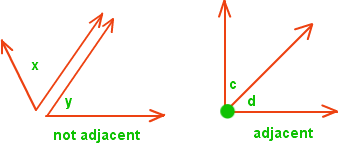Fig. 1. Fig. 2.

In the above figures, we have shown what types of angles are called adjacent angles. In fig. 2 angle c and angled are adjacent to each other because they have a common vertex and one common arm that is forming two different angles. In fig. 1 angle x and angle y are not adjacent angles because they do not have common arm and common vertex to form two different angles.

Still, if you are confused somewhere then we have one better example involving two pizza slices. When two pizza slices are placed next to each other in the box, the corners of both the slices are at the center of the box. In the whole pizza, there are so many other pairs of adjacent angles. Every slice of pizza has two possible different adjacent angles attached to one another.

Further, adjacent angles can be divided into two parts.

• 1. Complementary angles

• 2. Supplementary angles

• Two angles are said to be complementary when the sum of both the angles is 90° degrees. When two complementary angles are adjacent to each other then angle becomes a right angle.

Similarly, when two lines intersect and form four opposite angles, these angles are called opposite angles. Hence, complementary angles are also called opposite angles.

Two angles are said to be supplementary when the sum of both angles is 180° degrees. When two supplementary angles are adjacent to each other then angles are called supplementary angles.

Corresponding angles:

In corresponding angles, two lines are crossed by another line and the matching corners are called corresponding angles. Let discuss with the help of a figure.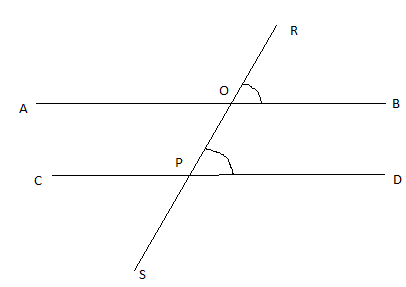fig.

In the above figure, AB and CD are parallel to each other to which RS is intersecting. The intersecting line is forming angles which are angle ROB and OPD. Now, Angle ROB and angle OPD are the corresponding angles. Remember that corresponding angles are also always equal to each other.

Below are some basic shapes through which we have tried to make you understand more about adjacent angles.
Triangle: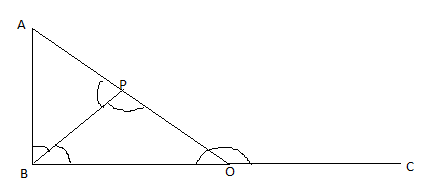In the above figure of the triangle, there are many examples of complementary angles and supplementary angles and we can elaborate it as that the angle ABP and the adjacent angle PBO can be combined to get an angle of 90 degrees to form a complementary angle. Moreover, the angle AOB and its adjacent angle AOC can be combined to form an angle of 180 degrees to be called as supplementary angles. Angle APB and angle BPO are also the examples of adjacent (complementary) angles in the above given triangle.

Square: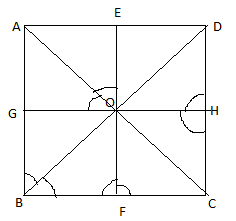In the figure of a square given above, there are many examples of complementary angles and supplementary angles and we can elaborate it as, the angle GBO and its adjacent angle FBO can be combined to form the angle of 90 degrees to form a complementary angle. Similarly, the angle GOA and its adjacent angle EOA can also be combined to form a complementary angle having the angle of 90 degrees. While the angle BFO and its adjacent angle CFO can be combined to form Supplementary angle (forming an angle of 180 degrees). Similarly, the angle CHO and its adjacent angle DHO can be combined to form the angle of 180 degrees to form a supplementary angle in the above given figure of a square.

How to find the missing adjacent angle?

We have discussed above about the adjacent angle with the help of examples. Now, we will discuss how to calculate the value of a adjacent angle through another given angle with the help of numerical. Let’s discuss-
For example - Find the values of x in the below figure.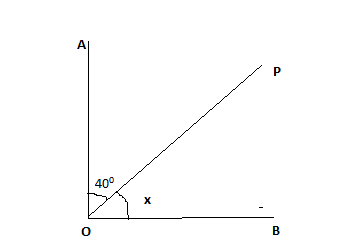Solution – In above figure, angle AOB = 90° (right angle)
Then, angle AOB + 40° = 90°
Angle AOB = 90° - 40° = 50°

Hence, we have calculated the value of missing adjacent angle.
But this is an example of complementary adjacent angles.
Let us take one example of supplementary angles.
For example – Find the value of y in the given figure: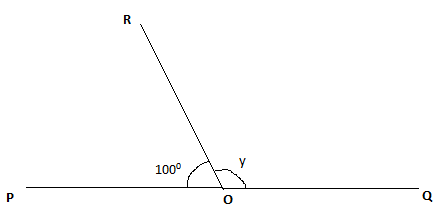In above figure, angle POQ = 180° (straight angle)
Then, angle ROP = 100° (given)
Angle ROQ = y
Angle y = angle POQ – angle POR
= 180° - 100°
= 80°
Hence, the value of y is calculated.

Vertically opposite Angles

When two lines intersect each other at one point and the angles opposite to each other are formed with the help of that two intersected lines, then the angles are called vertically opposite angles. These angles are always equal to each other.
You will understand this more clearly with the help of the figure given below: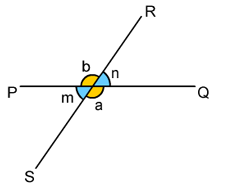In the above figure, lines PQ and RS intersect each other and form four different angles such as angle b, m, n, and a. In this case, angle m & n and angle b & a are vertically opposite to each other.

Now, you might be thinking that how vertically opposite angles are equal to each other. Let us discuss how vertically angles are opposite to each other.

Let us take the example of above given figure. In this,
m + b = 180° (linear pair of angles)
b + n = 180° (linear pair of angles)

Now, from the above equation, it is clear that m = n
Hence, it is proved that vertically opposite angles are equal.

How to find the missing vertical angle?

If two angles out of four are mentioned and you need to calculate the remaining two angles then have a look at the below example.
For example - Find the values of x and y in the figure below.
A R
P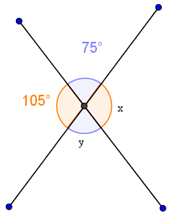B

Solution – In above figure, 75° + x = 180° (linear pair of angles)
Then, x = 180° - 75° = 105°
Similarly, 105° + y = 180° (linear pair of angles)
Then, y = 180° - 105° = 75°
Hence, the missing values are calculated.

Theoretical description of adjacent angles and vertical angles:

• 1. Adjacent angles - Adjacent angles are two angles that have common arm and common vertex.

•  Vertical angles - Two lines intersect each other and form angles. The opposite angles are called vertically opposite angles.

• 2. Adjacent angles - There are two types of adjacent angles.

• Vertical angles - Vertical angle has no types.